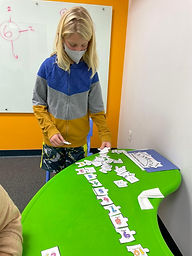Ms. Briana

Target 1​

Lesson Type:

Review

Number Operation

:

Integer Composition

Use place-value understandings to round whole numbers to the nearest ten or 100.

1:

Understand that rounding identifies the multiple of 10 or 100 that is closest to the given number.

2:

Understand that the previous place (one place smaller) is the key digit to evaluate to determine whether a number is rounded up or down.

3:

Use understanding of how to round two-digit numbers and expand the strategy to three-digit numbers.

2nd

Vocabulary:

Rounding, "Boss Baby" Method

Activities:

Students created rounding rollercoasters.

Students rolled dice and used the "Boss Baby" method and their roller coasters to round to the 10's place and 100's place.Home Exploration

Guiding Questions:Absent Students:

Ela, Blake?

Target 2

:

1:

Determine whether a given whole number in the range of one to 100 is a multiple of a given one-digit number.

2:

Recognize patterns in multiples, setting the foundation for developing computational fluency in multiplication.

4th

Vocabulary:

Factors, Multiples

Activities:

Students created factor germs on the large white board and worked together to determine factors.

Students began putting together a factor and multiple puzzle.Home Exploration

Guiding Questions:Target 3

:

Vocabulary:

Activities:Home Exploration